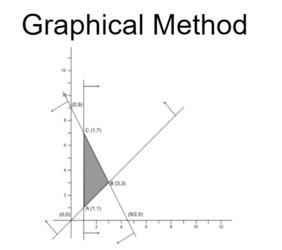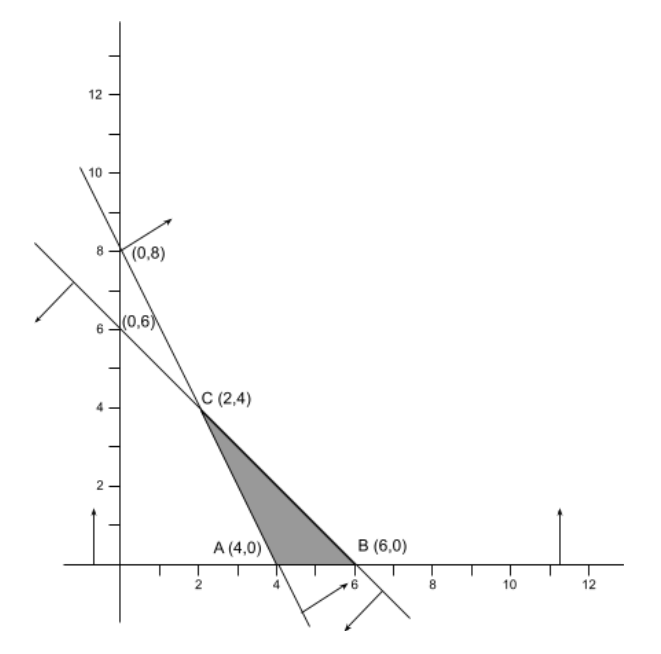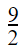Graphical Method of Solving Linear Programming ProblemsGraphical Solution of LP Problems

Graphical method of linear programming is used for solving LP problems by finding out the maximum or minimum point of the intersection between the objective function line and the feasible region on a graph.

The graphical method is used to optimize LP problems with two variables.

Feasible region:

The closed plain region obtained by the intersection of planes determined by a set of constraints in the LP problem is known as the feasible region.

The corner points of the feasible region are known as the vertices of the feasible region.

The values of the set of decision variables that satisfy the given constraints is the feasible solution.

Solution of a given LP Problem:

1. Formulate the problem using objective function and constraints.

2. Express inequalities as equations.

3. Plot graph from the points obtained from the equations.

4. Determine the valid side of each plane on the graph.

5. Identify the vertices of the feasible region.

6. Evaluate the value of objective function at each of the vertices of the feasible region.

7. Find the vertex at which the objective function attains the optimum (max. or min.) value.

8. Interpret the optimal value.

Exercise:

Find the feasible region determined by the following inequalities. Also, find the vertices of the region.

x + y ≤ 6

2x + y ≥ 8,

y ≥ 0

Solution:

The corresponding equations of the given inequalities are,

x + y = 6 ----(i)

2x + y = 8 ----(ii)

y = 0 ----(iii)

From equation (i),

When x=0, y=6 and

When y=0, x=6.

So, the boundary line (i) passes through (0,6) and (6,0).

Taking (0,0) as a test point for the inequality;

0+0 ≤ 6

or, 0 ≤ 6 (True)

∴ The half-plane determined by x + y ≤ 6 lies towards origin.

From equation (ii),

When x=0, y=8 and

When y=0, x=4.

So, the boundary line (ii) passes through (0,8) and (4,0).

Taking (0,0) as a test point for the inequality;

0+0 ≥ 8

or, 0 ≥ 8 (False)

∴ The half-plane determined by 2x + y ≥ 8 lies away from origin.

From equation (iii),

y=0  is the line parallel to X-axis.

∴ The half-plane determined by y ≥ 0 lies above the X-axis.

The feasible region determined by given inequalities is presented as the shaded region in the graph below:The vertices of the feasible region ABC are A (4,0), B (6,0) and C (2,4).

Exercise 2:

Maximize and Minimize:

F (x,y) = 6x + 9y

subject to,

2x + y ≤ 9

y ≥ x,

x ≥ 1

Solution:

Given objective function is, Z= 6x + 9y

The corresponding equations of the given inequalities are,

2x + y = 9 ----(i)

y = x ----(ii)

x = 1 ----(iii)

From equation (i),

When x=0, y=9 and

When y=0, x=So, the boundary line (i) passes through (0,9) and (,0).

Taking (0,0) as a test point for the inequality;

0+0 ≤ 9

or, 0 ≤ 9 (True)

∴ The half-plane determined by 2x + y ≤ 9 lies towards origin.

From equation (ii),

When x=0, y=0 and

When y=1, x=1.

So, the boundary line (ii) passes through (0,0) and (1,1).

Taking (1,0) as a test point for the inequality;

0 ≥ 1 (False)

∴ The half-plane determined by y ≥ x lies away from (1,0).

From equation (iii),

x=1 is the line parallel to y-axis.

∴ The half-plane determined by x ≥ 1 lies to the right of x=1.

The feasible region determined by given inequalities is presented as the shaded region in the graph below:The vertices of the feasible region ABC are A (1,1), B (3,3) and C (1,7).

Evaluation table for the objective function:

 Vertices: x y Objective Function: F (x,y) = 6x + 9y Remarks A (1,1) 1 1 6.1 + 9.1 = 15 Minimum B (3,3) 3 3 6.3 + 9.3 = 45 C (1,7) 1 7 6.1 + 9.7 = 69 Maximum

Hence, the maximum value is 69 at C (1,7) and the minimum value is 15 at A (1,1).

Exercise 3:

A factory turns out two articles A and B, each of which is processed to two machines X and Y. A requires two hours of X and 4 hours of Y; B requires 4 hours of X and 2 hours of Y

1.  If x is the number of A and y is the number ofB produced daily, write down two inequalities in x and y, noting that neither X nor Y can work more than 24 hours a day.

2. Assuming that all articles produced are sold, and each article A yields a profit of Rs. 60 and each article B yields a profit of Rs. 100, find how many of each article should be produced daily for maximum profit.

Solution:

Given question can be tabulated as follows:

 A (x) B (y) Limit X 2 hrs 4 hrs 24 hrs Y 4 hrs 2 hrs 24 hrs Profit Rs. 60 Rs. 100

For X, 2x + 4y ≤ 24

or,  x + 2y ≤ 12

For Y, 4x + 2y ≤ 24

or,  2x + y ≤ 12

Objective Function F(x,y) = 60x + 100y

Mathematical Model:

Max. F(x,y) = 60x + 100y

subject to,

x + 2y ≤ 12

2x + y ≤ 12

x,y ≥ 0

The corresponding equations of the given inequalities are,

x + 2y = 12 ----(i)

2x + y =12 ----(ii)

x,y  = 0 ----(iii)

From equation (i), x + 2y = 12

When x=0, y=6 and

When y=0, x=12

So, the boundary line (i) passes through (0,6) and (12,0).

Taking (0,0) as a test point for the inequality;

0+0 ≤ 12

or, 0 ≤ 12 (True)

∴ The half-plane determined by x + 2y ≤ 12 lies towards origin.

From equation (ii), 2x + y =12

When x=0, y=12 and

When y=0, x=6.

So, the boundary line (ii) passes through (0,12) and (6,0).

Taking (0,0) as a test point for the inequality;

0+0 ≤ 12

or, 0 ≤ 12 (True)

∴ The half-plane determined by 2x + y ≤12 lies towards the origin.

From equation (iii),

x=0  is the line parallel to Y-axis.

∴ The half-plane determined by x ≥ 0 lies on the right of the Y-axis.

y=0  is the line parallel to X-axis.

∴ The half-plane determined by y ≥ 0 lies above the X-axis.

The feasible region determined by given inequalities is presented as the shaded region in the graph below:The vertices of the feasible region OABC are O (0,0), A (6,0), B (4,4) and C (0,6).

Evaluation table for the objective function:

 Vertices: x y Objective Function: F (x,y) = 60x + 100y Remarks O (0,0) 0 0 60.0 + 100.0 = 0 A (6,0) 6 0 60.6 + 100.0 = 360 B (4,4) 4 4 60.4 + 100.4 = 640 Maximum C (0,6) 0 6 60.0 + 100.6 = 600
Hence, the maximum profit is Rs. 640 where 4 units of Article A and 4 units of Article B are produced.